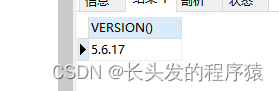# 一、数字函数

1、ABS(x) 返回x的绝对值

``SELECT ABS(-1);``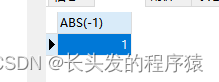2、AVG(expression) 返回一个表达式的平均值，expression 是一个字段

``SELECT AVG(score) FROM sc;``

结果：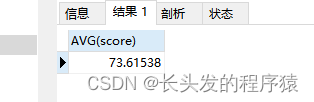3、CEIL(x)/CEILING(x) 返回大于或等于 x 的最小整数

``````SELECT CEIL(1.5);
SELECT CEILING(1.5); ``````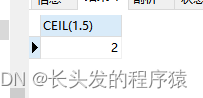4、FLOOR(x) 返回小于或等于 x 的最大整数

``SELECT FLOOR(1.5); ``5、EXP(x) 返回 e 的 x 次方

``SELECT EXP(3);``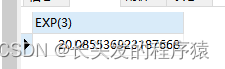6、GREATEST(expr1, expr2, expr3, …) 返回列表中的最大值

``SELECT GREATEST(3, 12, 34, 8, 25);``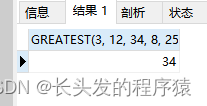7、LEAST(expr1, expr2, expr3, …) 返回列表中的最小值

``SELECT LEAST(3, 12, 34, 8, 25);``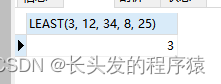8、LN 返回数字的自然对数

``SELECT LN(2);``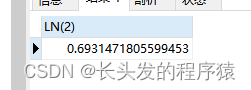9、LOG(x) 返回自然对数(以 e 为底的对数)

``SELECT LOG(20.085536923188);``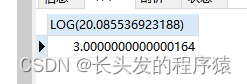10、MAX(expression)返回字段 expression 中的最大值

``SELECT MAX(score) FROM sc;``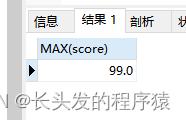11、MIN(expression)返回字段 expression 中的最小值

``SELECT MIN(score) FROM sc;``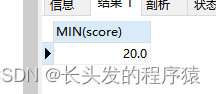12、POW(x,y)/POWER(x,y)返回 x 的 y 次方

``````SELECT POW(2,3);
SELECT POWER(2,3);``````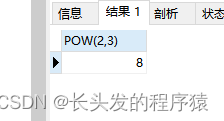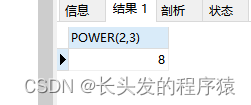13、RAND()返回 0 到 1 的随机数

``SELECT RAND();``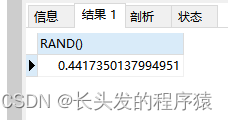14、ROUND(x)返回离 x 最近的整数

``SELECT ROUND(1.23456);``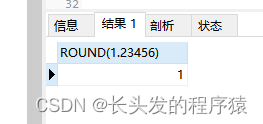15、SIGN(x)返回 x 的符号，x 是负数、0、正数分别返回 -1、0 和 1

``SELECT SIGN(-10);``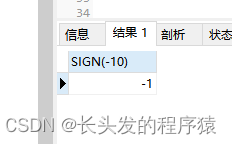16、SQRT(x)返回x的平方根

``SELECT SQRT(25);``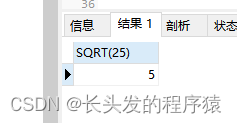17、SUM(expression)返回指定字段的总和

``SELECT SUM(score) FROM sc;``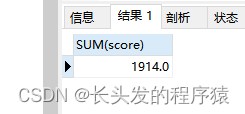18、TRUNCATE(x,y)返回数值 x 保留到小数点后 y 位的值（与 ROUND 最大的区别是不会进行四舍五入）

``SELECT TRUNCATE(1.23456,3);``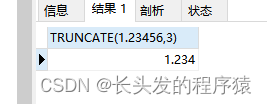# 二、字符串函数

1、返回字符串 s 的第一个字符的 ASCII 码

``SELECT ASCII('AB');``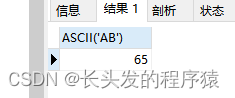2、LENGTH/CHAR_LENGTH(s)/CHARACTER_LENGTH(s)返回字符串 s 的字符数

``SELECT LENGTH('1234');``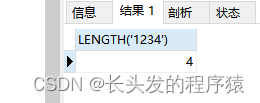3、CONCAT(s1,s2…sn)字符串 s1,s2 等多个字符串合并为一个字符串

``SELECT CONCAT('hel','llo');``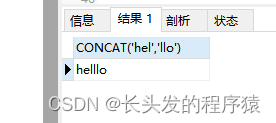4、FIND_IN_SET(s1,s2)返回在字符串s2中与s1匹配的字符串的位置

``SELECT FIND_IN_SET("c", "a,b,c,d,e");``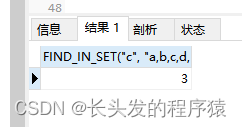5、FORMAT(x,n)函数可以将数字 x 进行格式化 “#,###.##”, 将 x 保留到小数点后 n 位，最后一位四舍五入

``SELECT FORMAT(250500.5634, 2); ``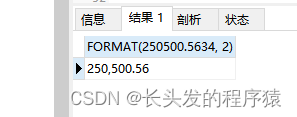6、INSERT(s1,x,len,s2)字符串 s2 替换 s1 的 x 位置开始长度为 len 的字符串

``SELECT INSERT("google.com", 1, 6, "runnob");``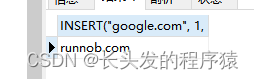7、LOCATE(s1,s)从字符串 s 中获取 s1 的开始位置

``SELECT LOCATE('st','myteststring');``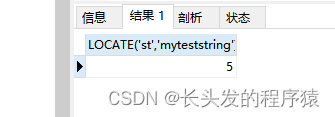8、LCASE(s)/LOWER(s)将字符串 s 的所有字母变成小写字母

``SELECT LOWER('RUNOOB');``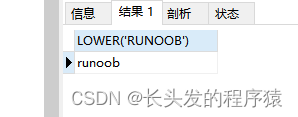9、UCASE(s)/UPPER(s)将字符串 s 的所有字母变成大写字母

``SELECT UCASE('runoob');``10、TRIM(s)去掉字符串 s 开始和结尾处的空格

``SELECT TRIM('    RUNOOB    ');``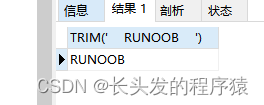11、LTRIM(s)去掉字符串 s 开始处的空格

``SELECT LTRIM('    RUNOOB    ');``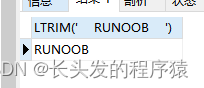12、RTRIM(s)去掉字符串 s 结尾处的空格

``SELECT RTRIM('    RUNOOB    ');``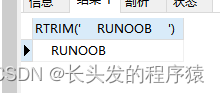13、SUBSTR/SUBSTRING(s, start, length)从字符串 s 的 start 位置截取长度为 length 的子字符串

``SELECT SUBSTR("RUNOOB", 2, 3);``
``SELECT SUBSTRING("RUNOOB", 2, 3);``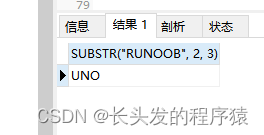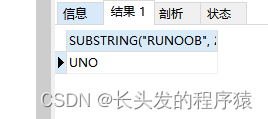14、POSITION(s1 IN s)从字符串 s 中获取 s1 的开始位置

``SELECT POSITION('b' in 'abc');``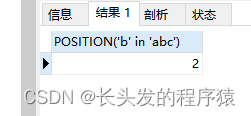15、REPEAT(s,n)将字符串 s 重复 n 次

``SELECT REPEAT('runoob',3);``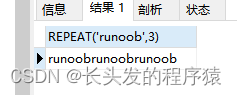16、REVERSE(s)将字符串s的顺序反过来

``SELECT REVERSE('abc');``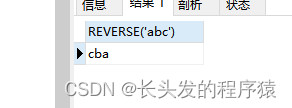17、STRCMP(s1,s2)比较字符串 s1 和 s2，如果 s1 与 s2 相等返回 0 ，如果 s1>s2 返回 1，如果 s1<s2 返回 -1

``SELECT STRCMP("runoob", "runoob");``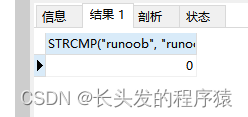# 三、日期函数

1、CURDATE()/CURRENT_DATE()返回当前日期

``````SELECT CURDATE();
SELECT CURRENT_DATE();``````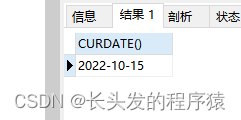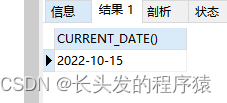2、CURRENT_TIME()/CURTIME()返回当前时间

``SELECT CURRENT_TIME();``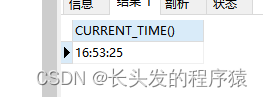3、CURRENT_TIMESTAMP()返回当前日期和时间

``SELECT CURRENT_TIMESTAMP();``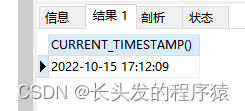``SELECT ADDDATE("2017-06-15", INTERVAL 10 DAY);``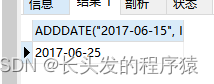``SELECT ADDTIME('2011-11-11 11:11:11', 5);``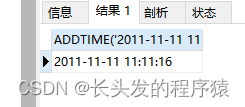6、DATE()从日期或日期时间表达式中提取日期值

``SELECT DATE("2017-06-15 11:11:16");``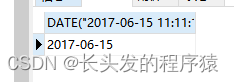7、DAY(d)返回日期值 d 的日期部分

``SELECT DAY("2017-06-15"); ``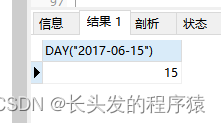8、DATEDIFF(d1,d2)计算日期 d1->d2 之间相隔的天数

``SELECT DATEDIFF('2001-01-01','2001-02-02'); ``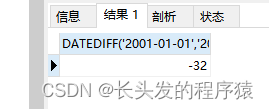9、DATE_FORMAT按表达式 f的要求显示日期 d

``SELECT DATE_FORMAT('2011.11.11 11:11:11','%Y-%m-%d %r');``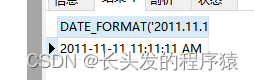10、DAYNAME(d)返回日期 d 是星期几，如 Monday,Tuesday

``SELECT DAYNAME('2011-11-11 11:11:11');``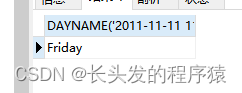11、DAYOFMONTH(d)计算日期 d 是本月的第几天

``SELECT DAYOFMONTH('2011-11-11 11:11:11');``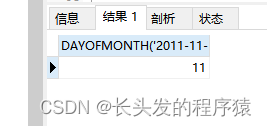12、DAYOFWEEK(d)日期 d 今天是星期几，1 星期日，2 星期一，以此类推

``SELECT DAYOFWEEK('2011-11-11 11:11:11');``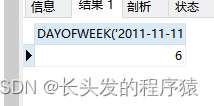13、DAYOFYEAR(d)计算日期 d 是本年的第几天

``SELECT DAYOFYEAR('2011-11-11 11:11:11');``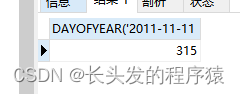14、EXTRACT(type FROM d)从日期 d 中获取指定的值，type 指定返回的值

``SELECT EXTRACT(MINUTE FROM '2011-12-13 14:15:16');``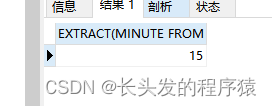15、UNIX_TIMESTAMP()得到时间戳

``SELECT UNIX_TIMESTAMP('2019-2-19');``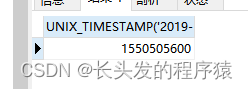# 四、MySQL高级函数

1、FROM_UNIXTIME()时间戳转日期

``SELECT IF(1>0,'yes','no');``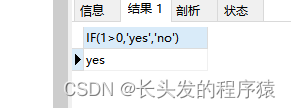2、CONV(x,f1,f2)返回 f1 进制数变成 f2 进制数

``SELECT CONV(13,10,2);``3、CURRENT_USER()/SESSION_USER()/SYSTEM_USER()/USER()返回当前用户

``SELECT CURRENT_USER();``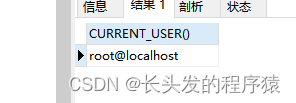4、DATABASE()返回当前数据库名

``SELECT DATABASE();``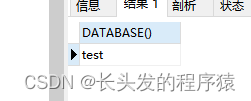5、VERSION()返回数据库的版本号

``SELECT VERSION();``